Name:    Quiz 3.1-3.2

Multiple Choice
Identify the choice that best completes the statement or answers the question.

Simplify the expression.

1.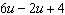a.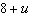c.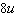b.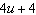d.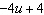2.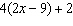a.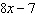c.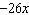b.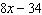d.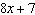3.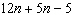a.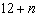c.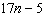b.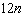d.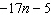4.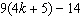a.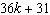c.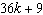b.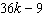d.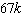Find the difference.

5.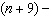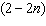a.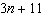c.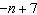b.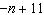d.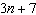6.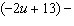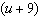a.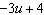c.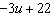b.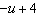d.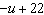Find the sum.

7.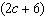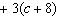a.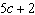c.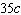b.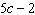d.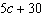8.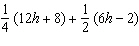a.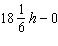c.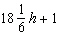b.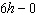d.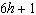Factor out the coefficient of the variable.

9.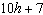a.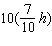c.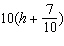b.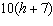d.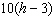10.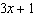a.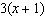c.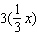b.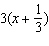d.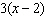11.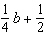a.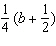c.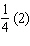b.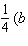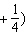d.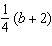12.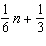a.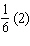c.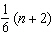b.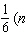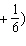d.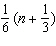Numeric Response

1.

After w weeks, a student has saved 25 + 8w dollars. How much money has the student saved after 15 weeks?

Identify the terms and like terms in the expression.

1.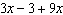2.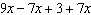3.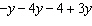Write an expression in simplest form that represents the perimeter of the polygon.

4.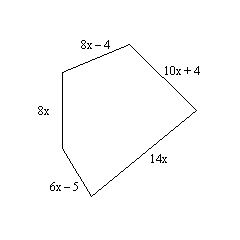5.

You and a friend decide to sell cookies to raise money for a local fundraiser. After d days, you have raised (6d + 7) dollars and your friend has raised (10d + 10) dollars. Write an expression that represents the total amount of money that you raised together.